# The Power of Desmos on the Digital SAT Math Section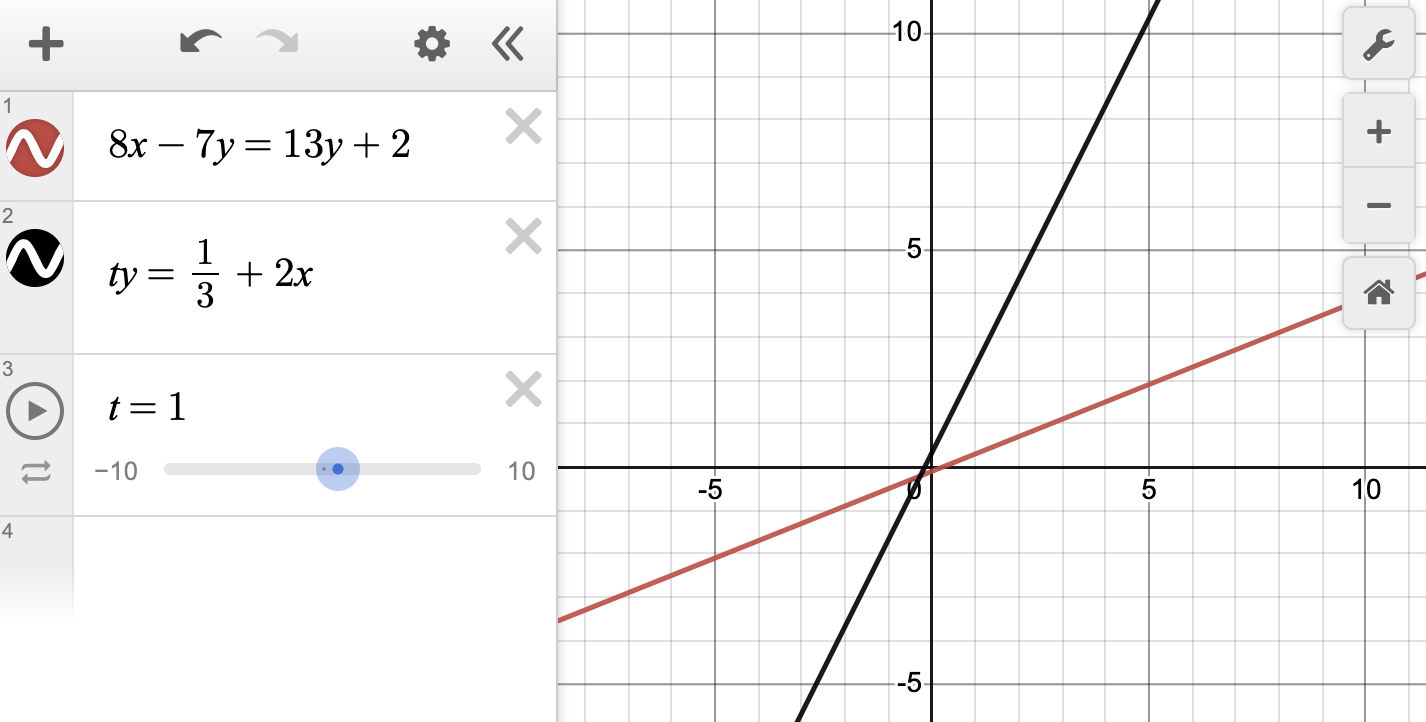The math content on the current SAT comprises two distinct sections: No Calculator and Calculator. For many students, having to answer an entire section of questions without a calculator poses significant challenges. But with the switch to the digital format, there will no longer be a No Calculator section. You read that correctly: on the new digital test, students will be allowed to use a calculator on all math questions.

Just as exciting is that the digital SAT will have Desmos built right into the testing software. In case you aren’t familiar with it, Desmos is a well-known digital graphing calculator that many high school students already use. And the news gets better: we at Method Learning have done a deep dive into all of the new digital SAT practice tests, and for the math section, we’ve discovered that roughly a third of all math questions can be solved using the Desmos calculator! What are the implications of this? So long as students know what to look for on the graph—the key to all questions that can be solved using Desmos—they will be able to use Desmos to identify the correct answer more quickly than they’d be able to do so by using traditional pencil-and-paper algebraic methods.

To see how using Desmos can save time (and hopefully even some stress!), let’s analyze a few sample questions similar to what students will see on the new digital math section.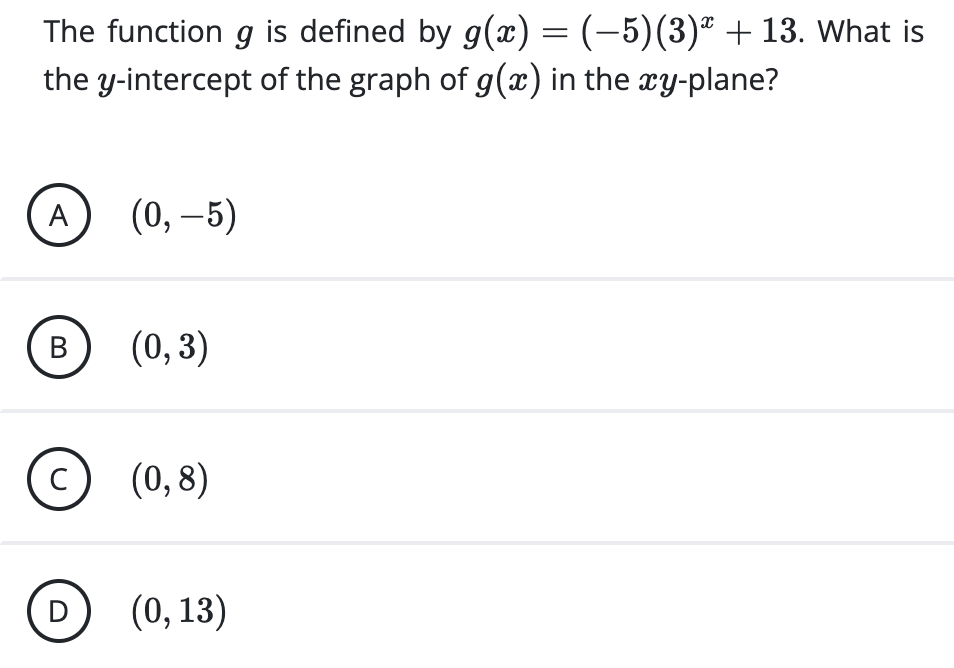We might know how to solve this question using algebra, and that’s great, but let’s talk about how to solve it by using Desmos, an approach that will likely be quicker than any other method for most students on this question. The y-intercept is where the graph crosses the y-axis, so if we type the function g(x) into Desmos, it’ll be easy to find the answer.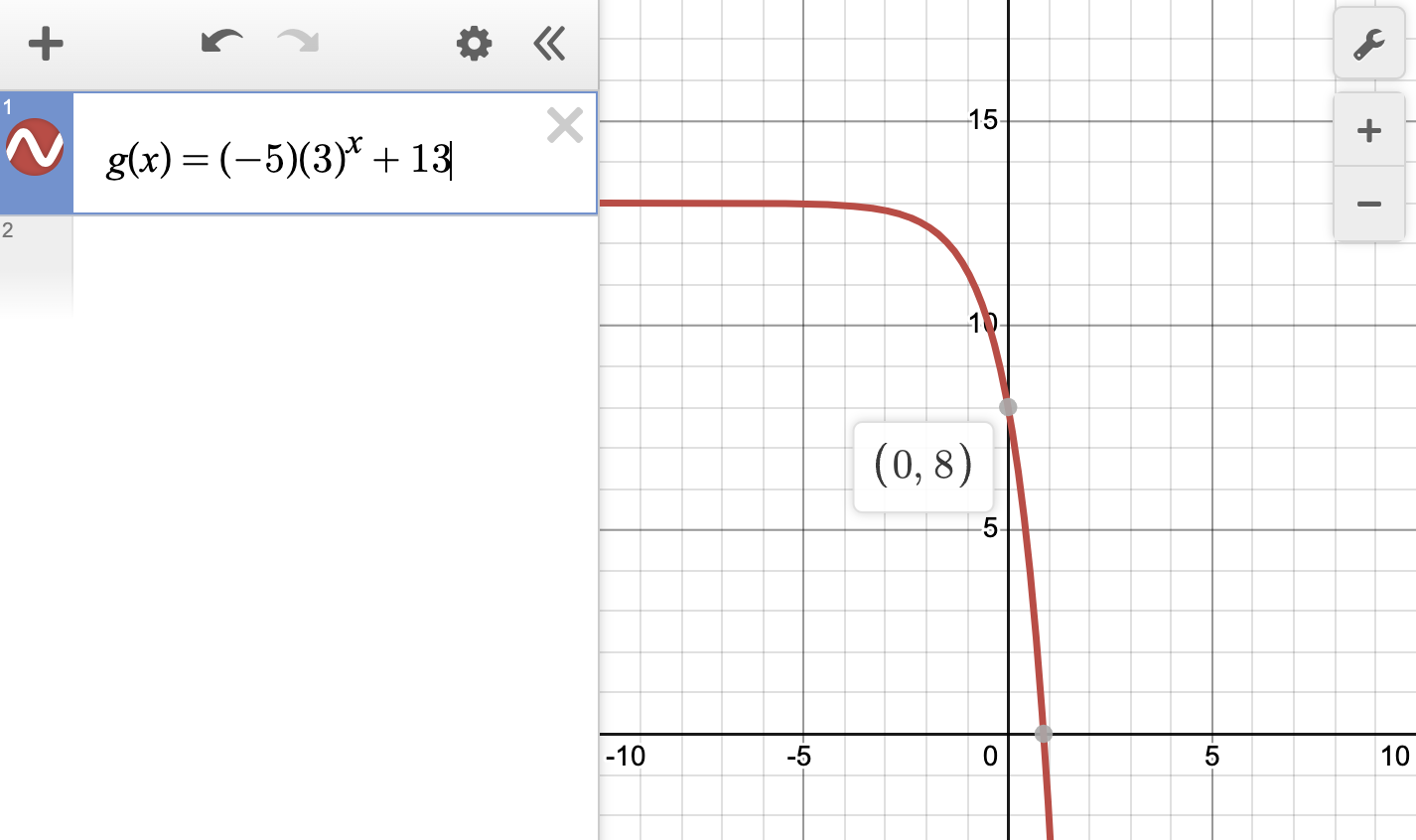So our answer is C. Note: you can hover your mouse over the y-intercept (or click on it) to get Desmos to reveal the point (0, 8) as we see in the above image.

Desmos really shines in problems like this next one, in which we’re missing a constant and need to find its value.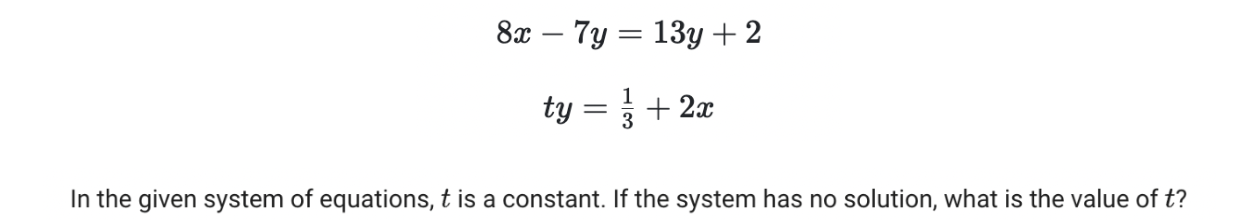How can we find t ? As a matter of fact, what are we even supposed to be doing in this problem? As we mentioned earlier, it’s all about knowing what to look for in Desmos. But before we go there, let’s discuss the basic principles underlying this problem. The important phrase is “the system has no solution.” For any system of equations—a fancy phrase that applies when we’re examining two equations simultaneously—a solution is a point where the two equations’ graphs intersect. But if there’s no solution, as is the case here, then the graphs don’t intersect. So we just need to find what value of t makes this happen. Plugging both equations into Desmos gives us the following.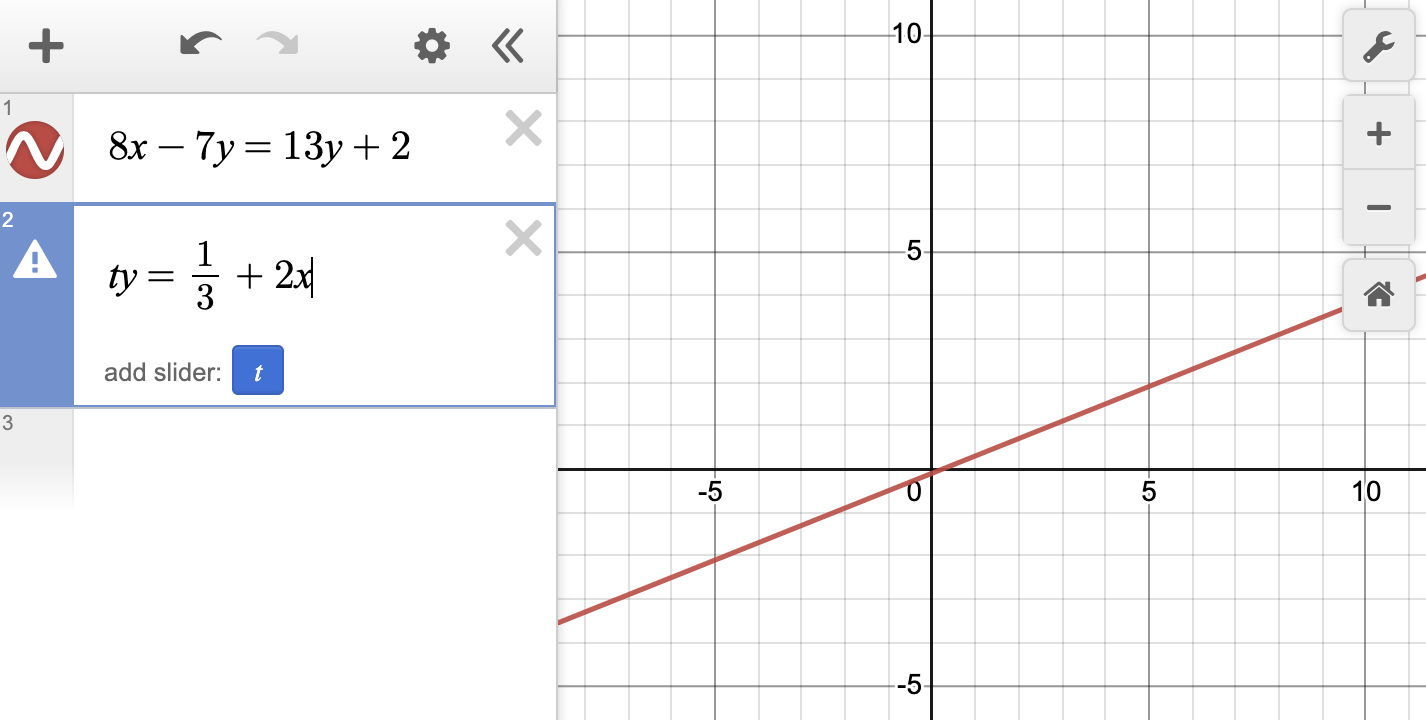We’ll want to click the blue “t” button to add a slider to manipulate the value of t.Clearly, if t = 1, the lines still intersect. Remember, we want the lines to have no intersection point. This is the cool part: we can drag the blue slider to change the value of t until we get the lines to be parallel: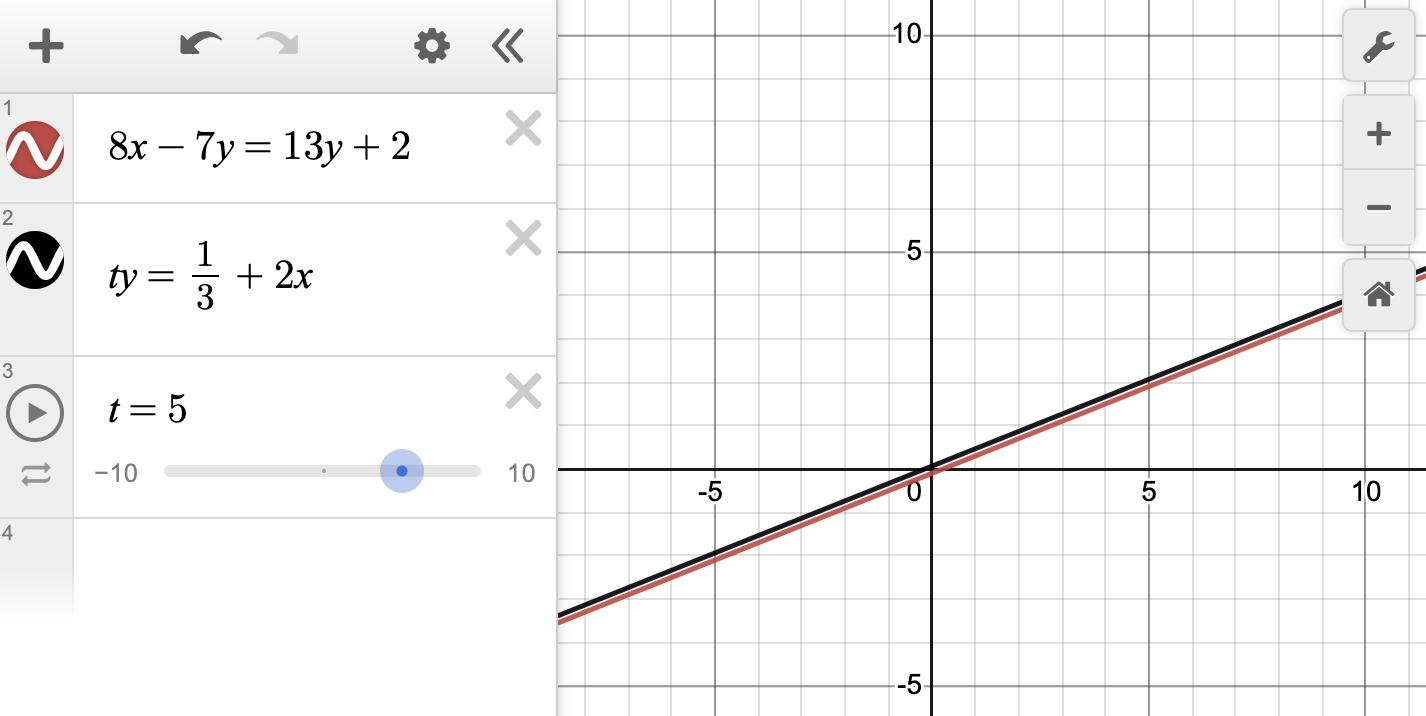If we need to zoom in on the graph to see this more clearly, Desmos lets us do that, too: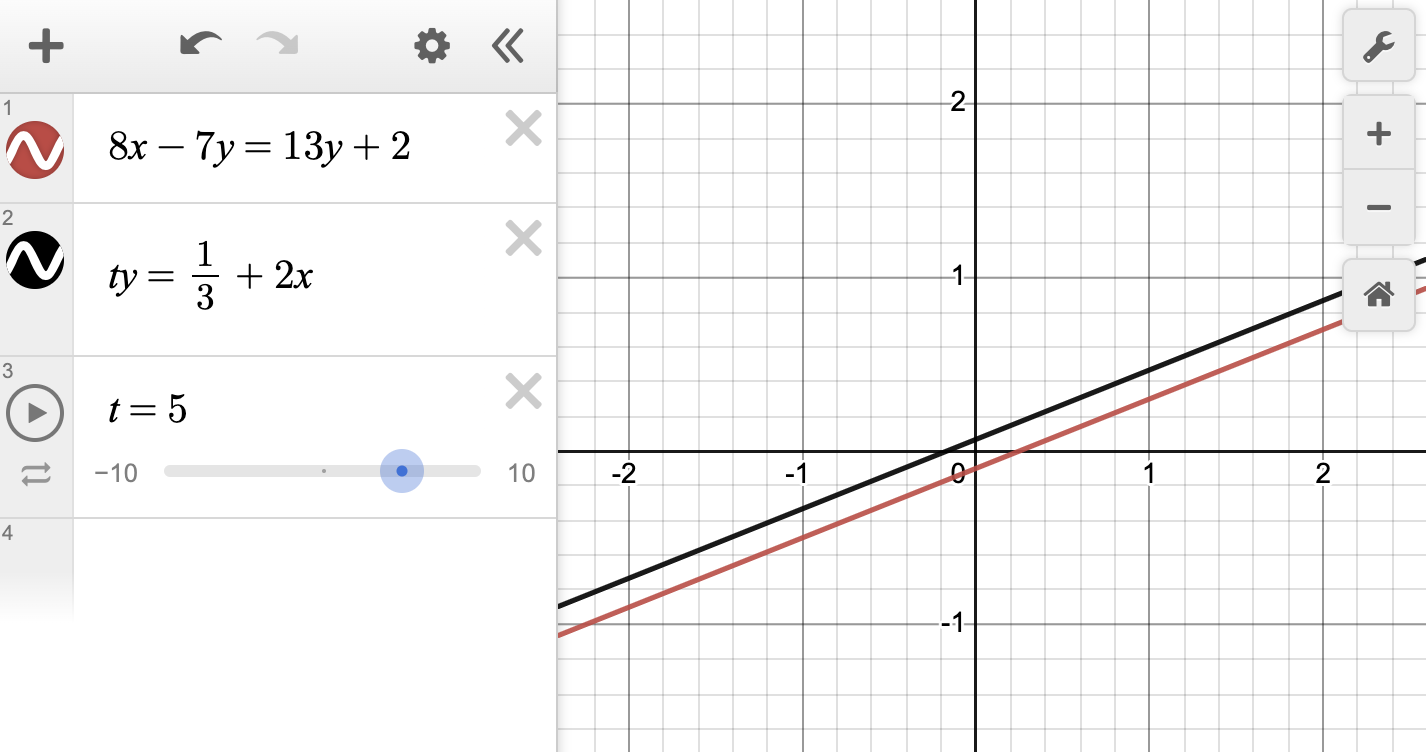So our final answer here is that t = 5. That probably seemed like a long problem, but if you know what you’re looking for in Desmos, you could probably solve it in just about 30 seconds! At this point, it should be clear that Desmos is an extraordinarily powerful tool that we’ll want to take advantage of on the SAT!

Let’s do just one more problem.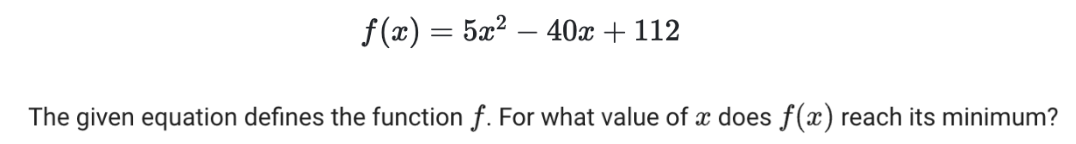The minimum refers to the lowest point that the graph of this quadratic equation reaches. So we’ll simply type the equation into Desmos as we see it and we’ll find our answer (we’ll have to zoom out a little or drag the graph up to find this minimum).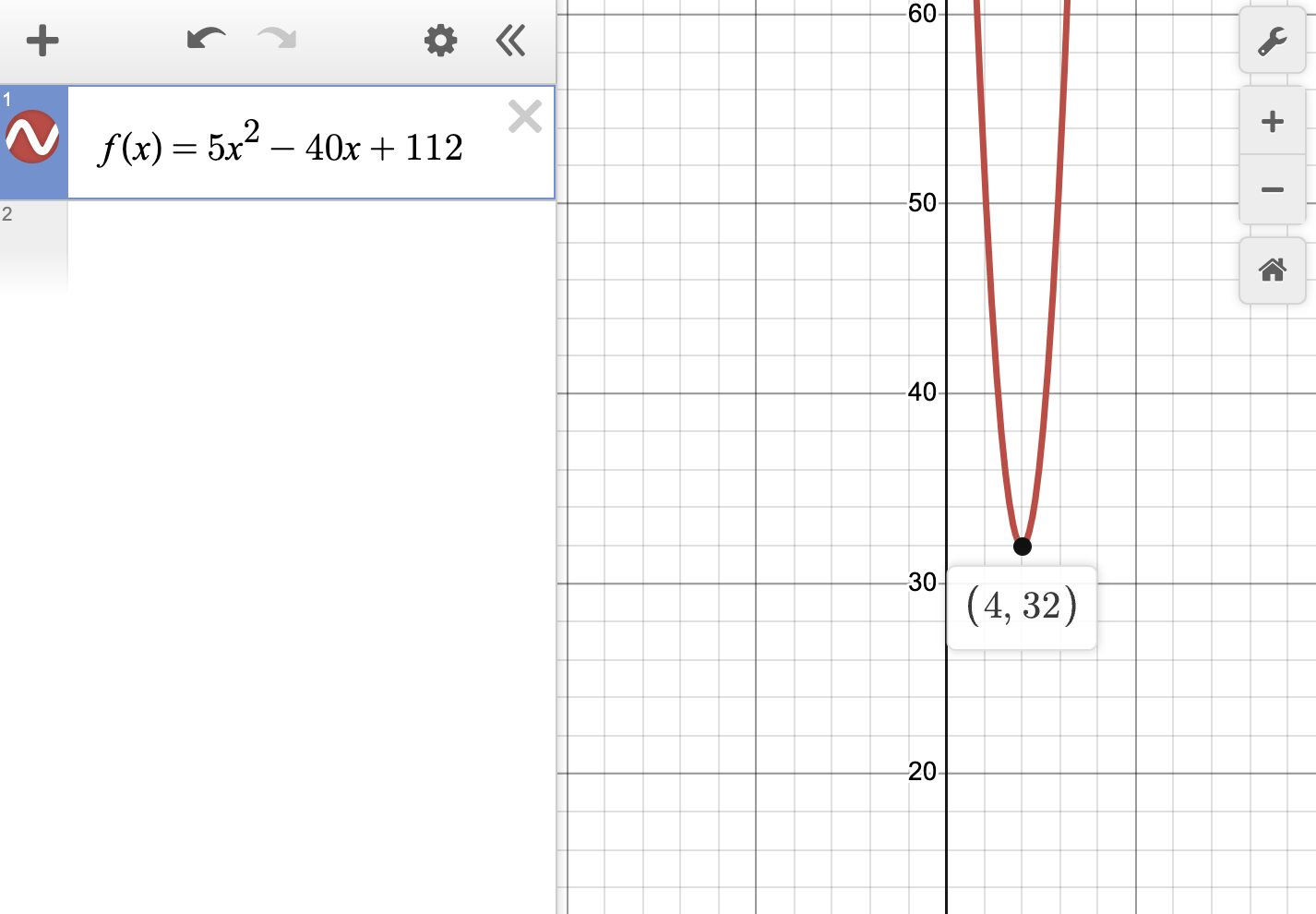So the minimum is (4, 32), but because the question asks specifically for the x-value at this minimum, we’ll want just the point’s x-coordinate: 4. That’s our answer!

With just these three examples, you should be convinced of the power of using Desmos to answer questions correctly on the digital SAT. In general, questions that present a function or that refer to a graph not given in the question can be solved using Desmos. Once again, the key to these problems is knowing what to look for, but with a little practice, students can master solving them, opening up opportunities to achieve higher scores. Of course, there are ways to answer these questions without a calculator, but even a basic facility with Desmos will allow most students to move through these problems—and many others—with more speed and confidence than they’d have while using almost any other strategy. We encourage all students who are planning to take the digital SAT to begin their practice with Desmos today to become more familiar with its features. Doing so will give them a huge advantage when the time comes for them to take the test.

To access digital SAT® information from the test creator itself, visit the College Board website. There, you’ll find more detail about the exam specifications, test registration, and official practice resources.

We’ve also produced several blog posts analyzing what students can expect to see on the digital SAT®.

Grammar Deep Dive